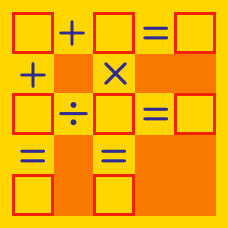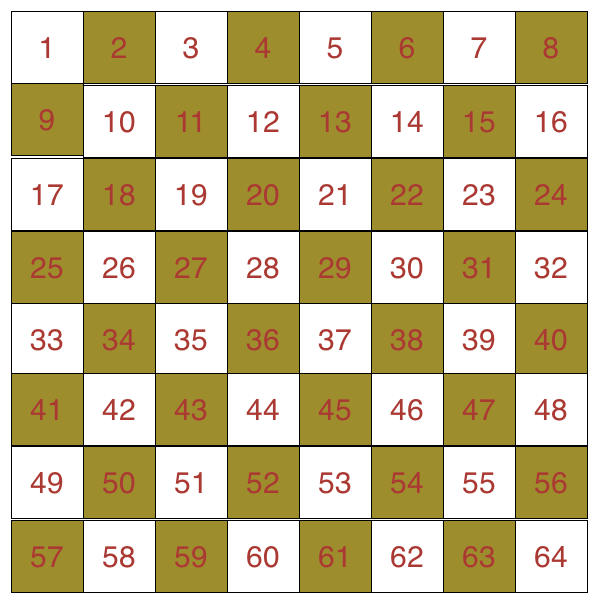Logic

# Arithmetic Puzzles: Level 1 ChallengesThe numbers 1 to 64 are written in order on the chessboard above. What is the sum of the numbers in the yellow squares minus the sum of the numbers in the white squares?

$\large (6 \, \square \, 2) \, \square \, (3 \, \square \, 4) \, \square \, (6\, \square \, 2) = 25$

Which of these options are the appropriate mathematical operators for the five squares in the above equation?

Bonus: Can you find other operators that work?

$\begin{array}{ccccc} & & & X & Y&Z\\ + & & & X & Z & Y \\ \hline & & & Y & Z & X\\ \end{array}$

Let $$X,Y$$ and $$Z$$ be three distinct single digits satisfying the cryptogram above with $$X\ne 0$$. Find the value of the three digit integer, $$\overline{XYZ}$$.

If every whole number from 1 to 1000 inclusive is written down, then which digit is written the least?A newspaper is printed on several sheets of paper and then folded down the middle. Each piece of paper will thus contain four pages.

On glancing through the newspaper whose back page is 28, you noticed that a sheet is missing. One of the missing pages is page number 8. What are the other three missing pages?

Write your answer in the increasing order of the number of pages. For example, if you think that pages 4, 11, and 25 are missing, then your answer will be 41125.

×

Problem Loading...

Note Loading...

Set Loading...